# ignore.c File Reference

groups and ignore command and functions. More...

`#include <stdlib.h>`
`#include <unistd.h>`
`#include <ctype.h>`
`#include <pcre.h>`
`#include <sys/mman.h>`
`#include "global.h"`
`#include "interface.h"`
`#include "waa.h"`
`#include "est_ops.h"`
`#include "helper.h"`
`#include "warnings.h"`
`#include "direnum.h"`
`#include "ignore.h"`
`#include "url.h"`

Include dependency graph for ignore.c: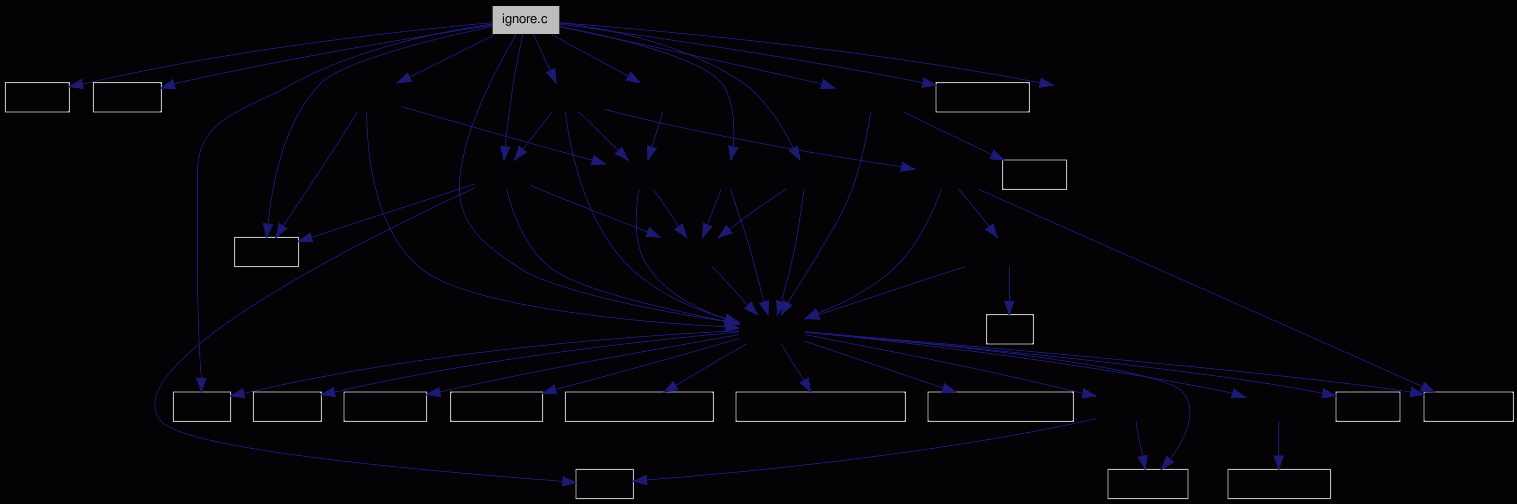Go to the source code of this file.

## Defines

#define RESERVE_IGNORE_ENTRIES   (4)

## Functions

int ign___translate_bracketed_expr (char *end_of_buffer, char **src, char **dest)
Processes a character class in shell ignore patterns.
int ign__compile_pattern (struct ignore_t *ignore)
Compiles the given pattern for use with `PCRE`.
int ign___init_pattern_into (char *pattern, char *end, struct ignore_t *ignore)
Does all necessary steps to use the given `ignore_t` structure.
-.
int ign___compare_dev (struct sstat_t *st, struct ignore_t *ign)
Compares the given `sstat_t` st with the device ignore pattern ign.
int ign___new_group (struct ignore_t *ign, struct grouping_t **result)
Loads the grouping definitions, and stores them via a grouping_t.
int ign__is_ignore (struct estat *sts, int *is_ignored)
-.
int ign__save_ignorelist (char *basedir)
Writes the ignore list back to disk storage.
int ign__new_pattern (unsigned count, char *pattern[], char *ends, int user_pattern, int position)
Adds a list of new ignore patterns to the internal list.
int ign___parse_position (char *arg, int *position, int *advance)
Parses the optional position specification.
int ign___test_single_pattern (struct estat *sts)
int ign___test_all_patterns (struct estat *sts)
int ign__print_group_stats (FILE *output)
-.
int ign__work (struct estat *root UNUSED, int argc, char *argv[])
-.
int ign__rign (struct estat *root UNUSED, int argc, char *argv[])
-.

## Variables

apr_hash_t * ign___groups = NULL
int ign__max_group_name_len = 6
The length of the longest group name, used for status.
static const char ign_header_str [] = "%u"
Header definition - currently only number of entries.
static const char ign__group_take [] = "take"
static const char ign__group_ign [] = "ignore"
const char ign___parm_delimiter = ','
int max_ignore_entries = 0
For how many grouping patterns memory is allocated.
int used_ignore_entries = 0
How many grouping patterns are actually used.
static struct ignore_tignore_list = NULL
Allocated array of grouping patterns.
static char * memory
Place where the patterns are mmap()ed.
static const char pcre_prefix [] = "PCRE:"
The various strings that define the pattern types.
static const char dev_prefix [] = "DEVICE:"
static const char inode_prefix [] = "INODE:"
static const char norm_prefix [] = { '.', PATH_SEPARATOR, 0 }
static const char wildcard_prefix [] = { PATH_SEPARATOR, '*', '*', 0 }
static const char abs_shell_prefix [] = { PATH_SEPARATOR, 0 }

## Detailed Description

groups and ignore command and functions.

Definition in file ignore.c.

## Define Documentation

 #define RESERVE_IGNORE_ENTRIES   (4)

Definition at line 614 of file ignore.c.

Referenced by ign__new_pattern().

## Function Documentation

 int ign___compare_dev ( struct sstat_t * st, struct ignore_t * ign ) ` [inline]`

Compares the given `sstat_t` st with the device ignore pattern ign.

Does the less-than, greater-than and/or equal comparision.

Definition at line 1368 of file ignore.c.

References sstat_t::dev, ignore_t::has_minor, ignore_t::major, and ignore_t::minor.

Referenced by ign__is_ignore().

Here is the caller graph for this function: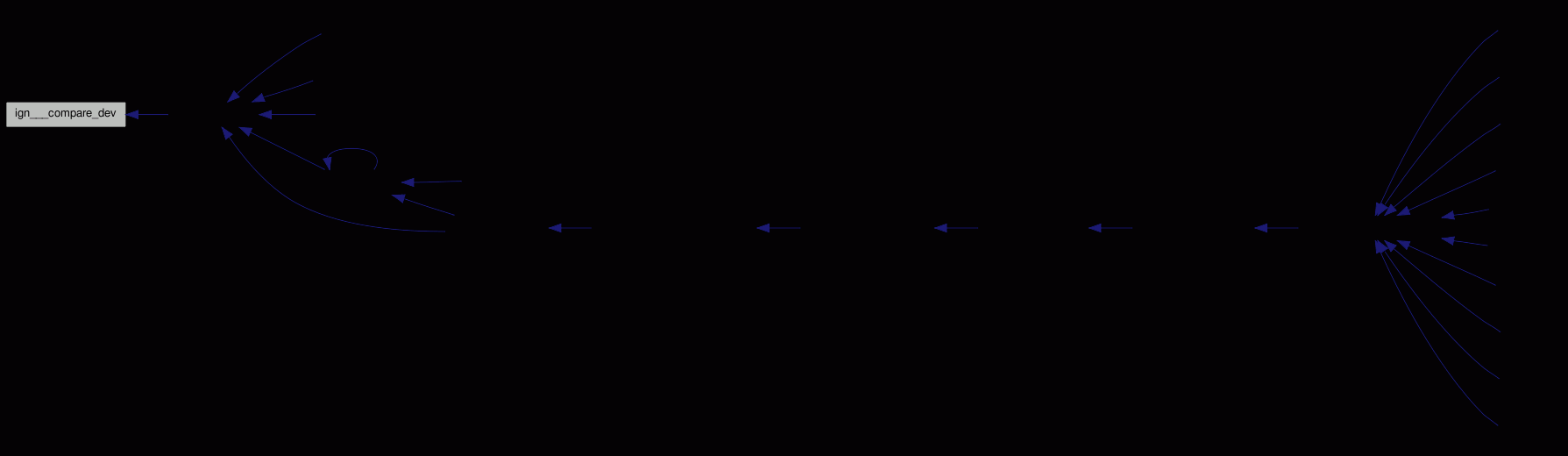int ign___init_pattern_into ( char * pattern, char * end, struct ignore_t * ignore )

Does all necessary steps to use the given `ignore_t` structure.

Definition at line 938 of file ignore.c.

Referenced by ign__new_pattern().

Here is the call graph for this function: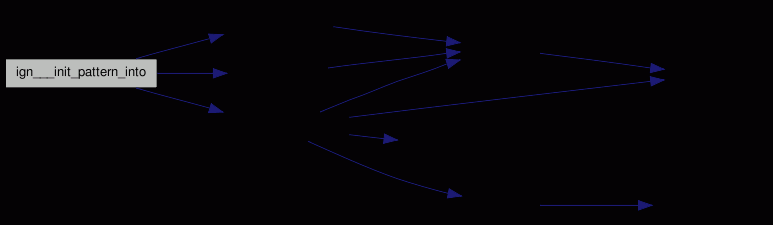Here is the caller graph for this function: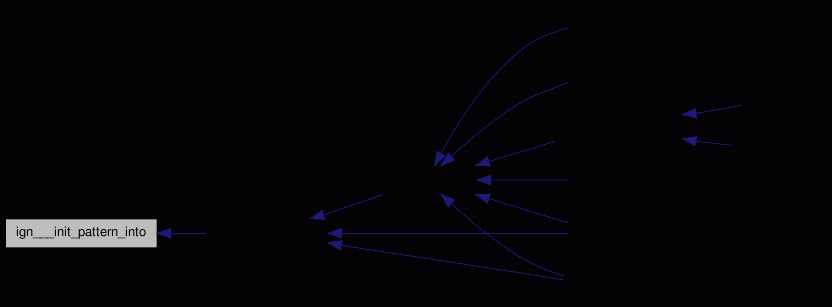int ign___load_group ( struct ignore_t * ign )

Loads the grouping definitions, and stores them via a grouping_t.

Definition at line 1429 of file ignore.c.

Referenced by ign__is_ignore().

Here is the call graph for this function:Here is the caller graph for this function: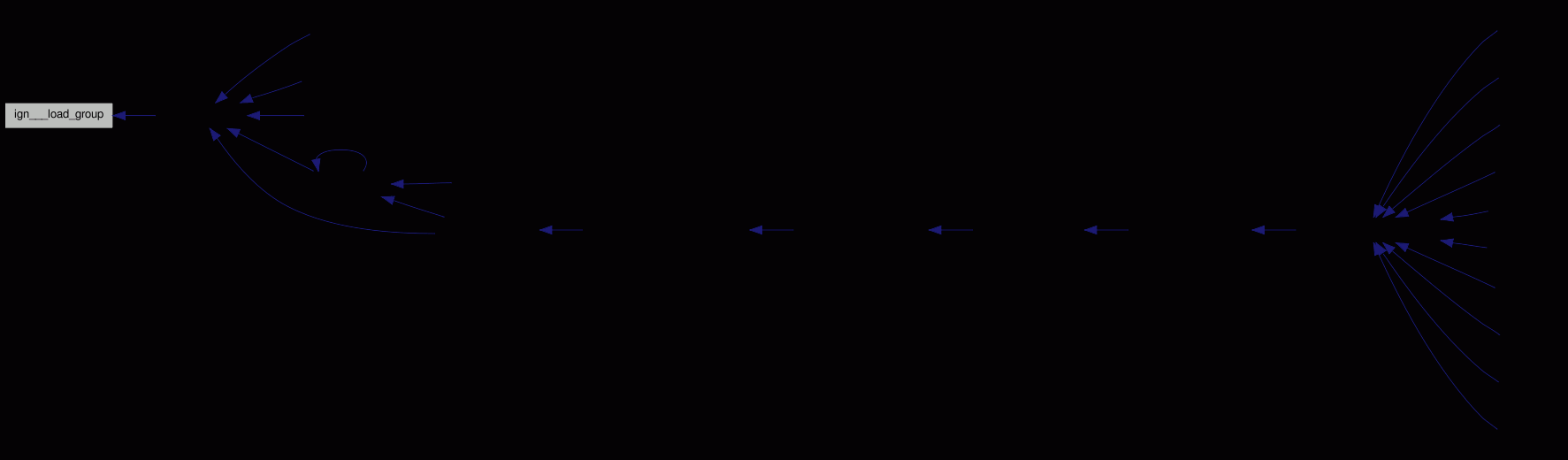int ign___new_group ( struct ignore_t * ign, struct grouping_t ** result )

Definition at line 1390 of file ignore.c.

Here is the call graph for this function: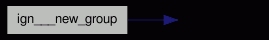Here is the caller graph for this function: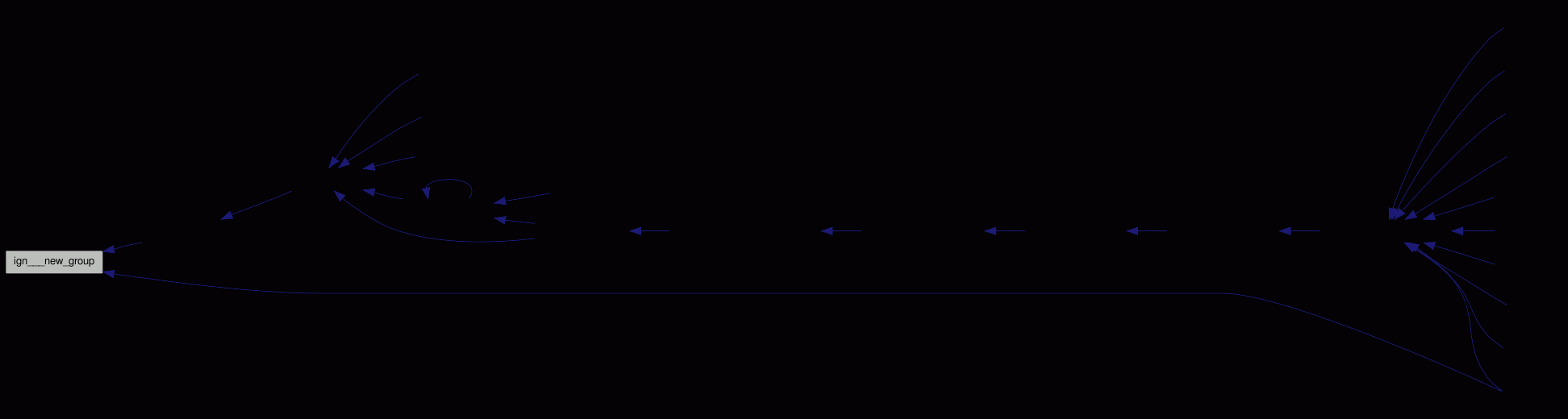int ign___parse_position ( char * arg, int * position, int * advance )

Parses the optional position specification.

Definition at line 1901 of file ignore.c.

Referenced by ign__rign(), and ign__work().

Here is the caller graph for this function: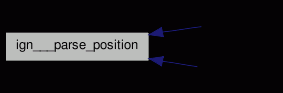int ign___test_all_patterns ( struct estat * sts )

Definition at line 1955 of file ignore.c.

Referenced by ign__work().

Here is the call graph for this function: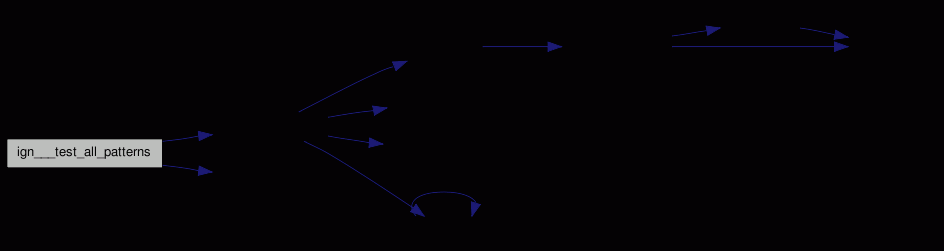Here is the caller graph for this function: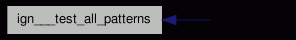int ign___test_single_pattern ( struct estat * sts )

Definition at line 1935 of file ignore.c.

Referenced by ign__work().

Here is the call graph for this function: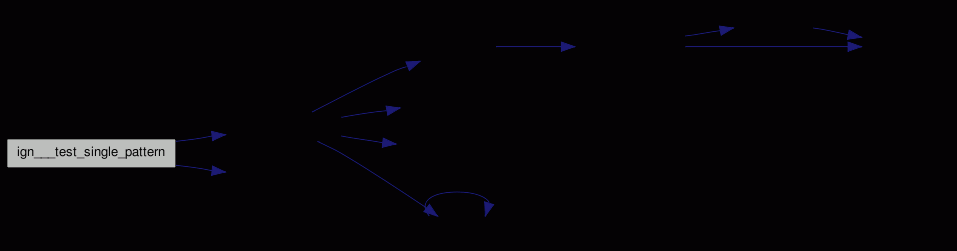Here is the caller graph for this function: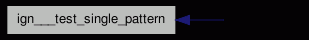int ign___translate_bracketed_expr ( char * end_of_buffer, char ** src, char ** dest )

Processes a character class in shell ignore patterns.

Definition at line 651 of file ignore.c.

References STOPIF, and STOPIF_CODE_ERR.

Referenced by ign__compile_pattern().

Here is the caller graph for this function: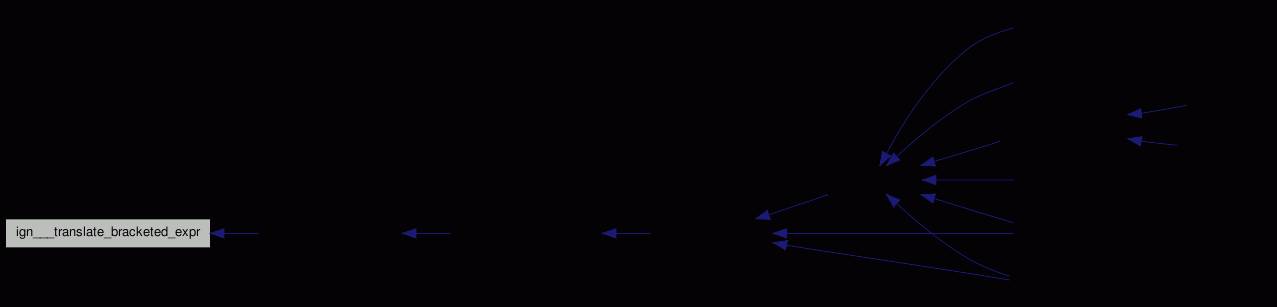int ign__compile_pattern ( struct ignore_t * ignore )

Compiles the given pattern for use with `PCRE`.

Definition at line 718 of file ignore.c.

Referenced by ign___init_pattern_into().

Here is the call graph for this function: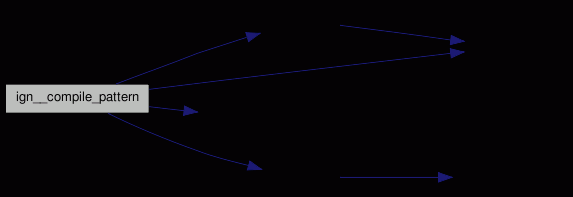Here is the caller graph for this function: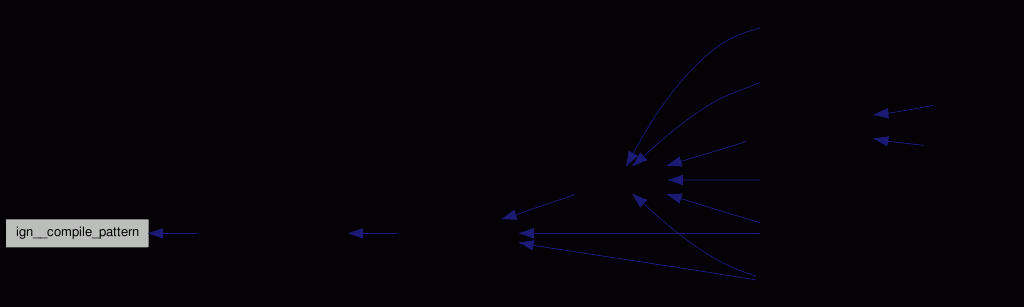int ign__is_ignore ( struct estat * sts, int * is_ignored )

-.

Tells whether the given entry is to be ignored.

Searches this entry for a take/ignore pattern.

If a parent directory has an ignore entry which might be valid for this directory (like **/*~), it is mentioned in this directory, too - in case of something like dir/a*/b*/* a path level value is given.

As we need to preserve the _order_ of the ignore/take statements, we cannot easily optimize. is_ignored is set to +1 if ignored, 0 if unknown, and -1 if on a take-list (overriding later ignore list).

sts must already have the correct estat::st.mode bits set.

Definition at line 1612 of file ignore.c.

Referenced by au__action(), prp__s_work(), st__status(), waa__build_tree(), and waa__update_dir().

Here is the call graph for this function: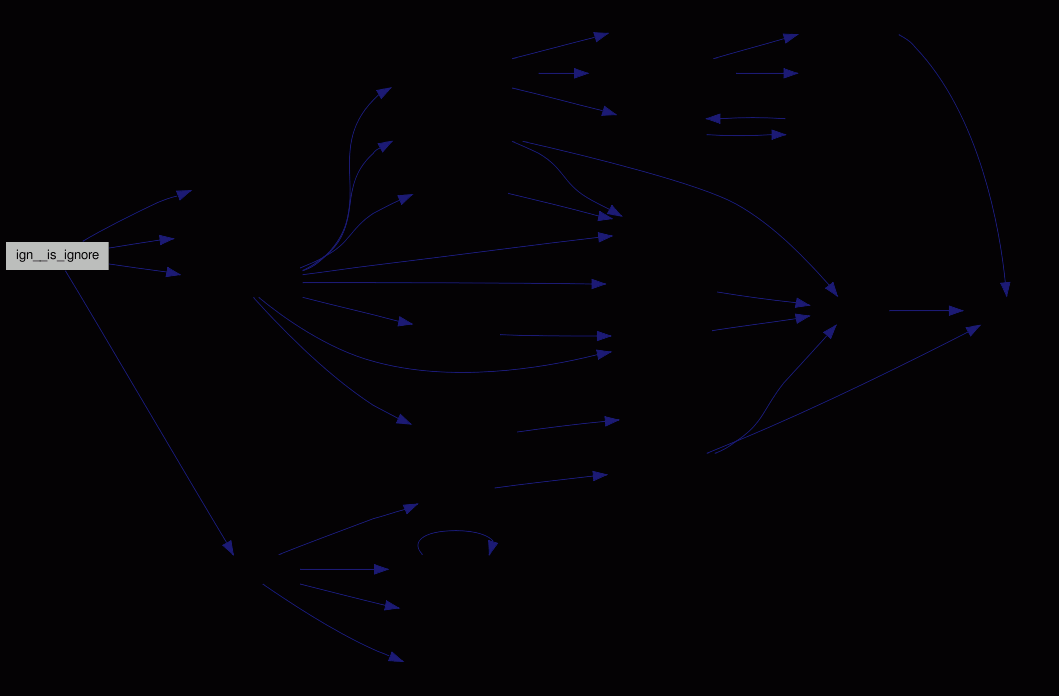Here is the caller graph for this function: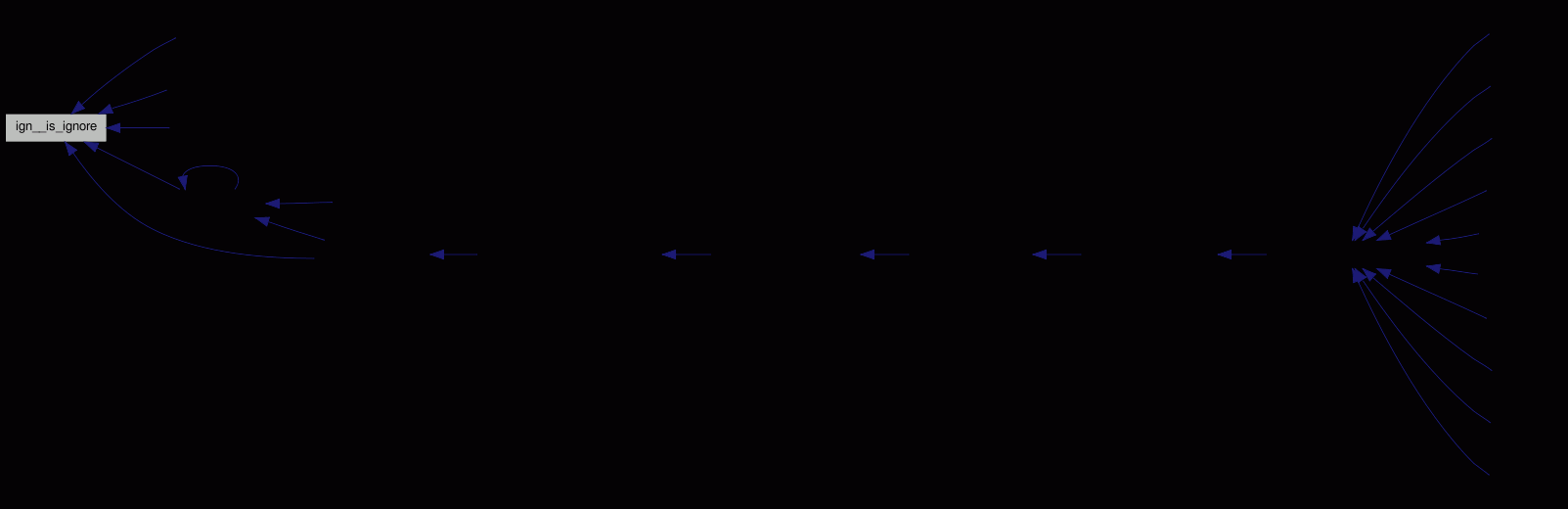int ign__load_list ( char * dir )

-.

Loads the ignore list from the WAA.

Definition at line 1282 of file ignore.c.

Referenced by au__prepare_for_added(), ci__work(), df__work(), ign__rign(), ign__work(), and st__work().

Here is the call graph for this function: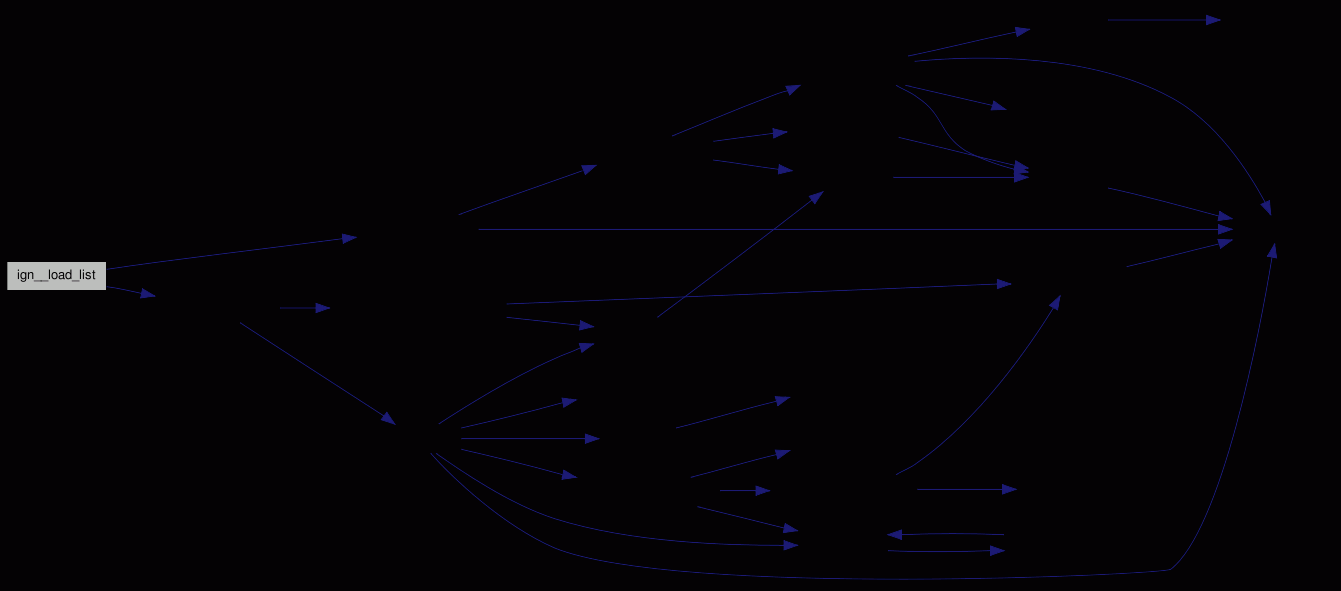Here is the caller graph for this function: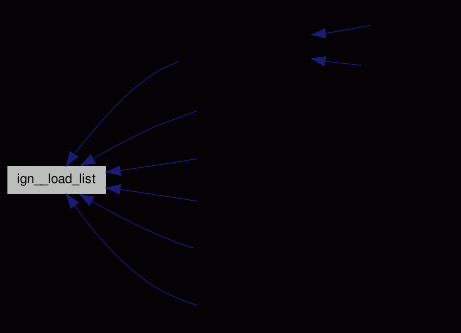int ign__new_pattern ( unsigned count, char * pattern[], char * ends, int user_pattern, int position )

Adds a list of new ignore patterns to the internal list.

Definition at line 1807 of file ignore.c.

Referenced by ign__load_list(), ign__rign(), and ign__work().

Here is the call graph for this function: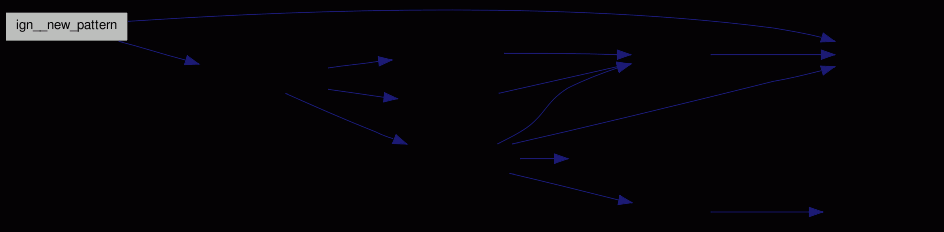Here is the caller graph for this function: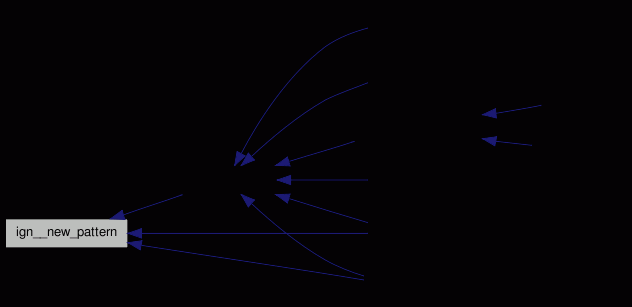int ign__print_group_stats ( FILE * output )

-.

Print the grouping statistics.

Definition at line 1983 of file ignore.c.

Referenced by ign__work(), and st__work().

Here is the call graph for this function: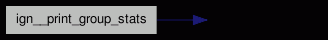Here is the caller graph for this function: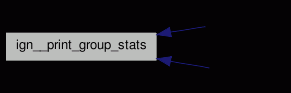int ign__rign ( struct estat *root UNUSED, int argc, char * argv[] )

-.

Relativizes the given paths, and stores them.

Definition at line 2155 of file ignore.c.

Here is the call graph for this function: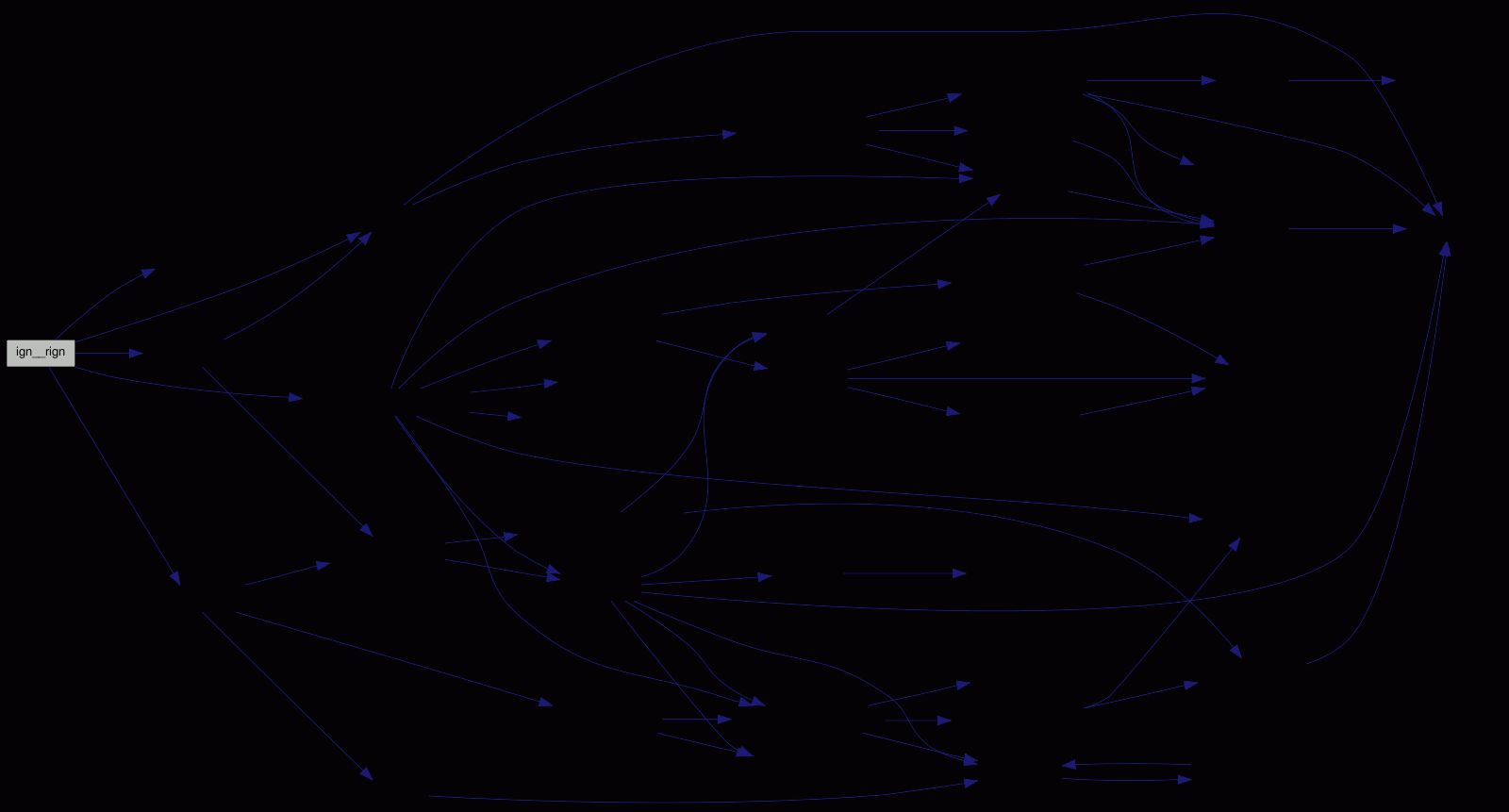int ign__save_ignorelist ( char * basedir )

Writes the ignore list back to disk storage.

Definition at line 1739 of file ignore.c.

Referenced by ign__rign(), and ign__work().

Here is the call graph for this function: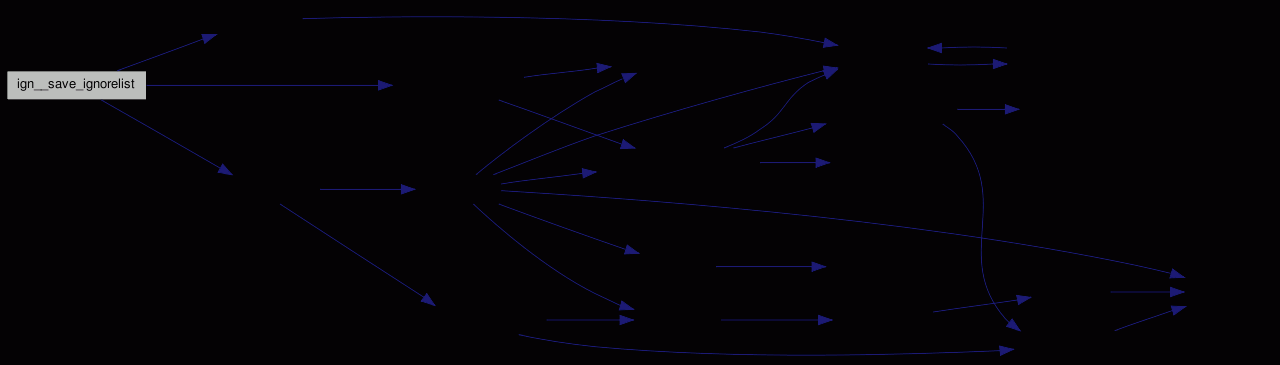Here is the caller graph for this function: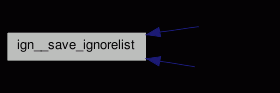int ign__work ( struct estat *root UNUSED, int argc, char * argv[] )

-.

This is called to append new ignore patterns.

Definition at line 2012 of file ignore.c.

Here is the call graph for this function: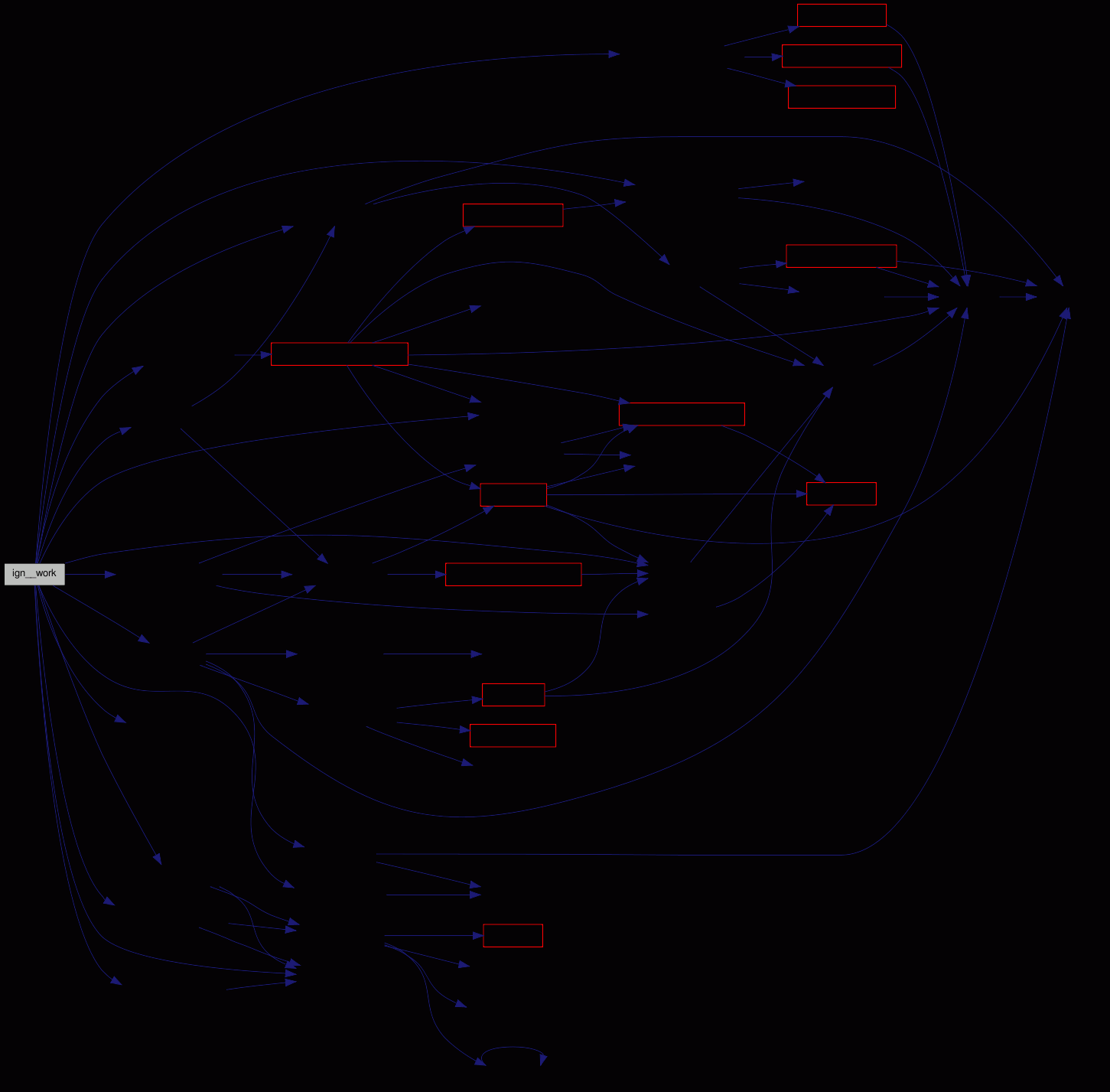## Variable Documentation

 const char abs_shell_prefix[] = { PATH_SEPARATOR, 0 }` [static]`

Definition at line 645 of file ignore.c.

Referenced by ign___init_pattern_into().

 const char dev_prefix[] = "DEVICE:"` [static]`

Definition at line 639 of file ignore.c.

Referenced by ign___init_pattern_into().

 const char ign___parm_delimiter = ','

Definition at line 621 of file ignore.c.

Referenced by ign___init_pattern_into().

 const char ign__group_ign[] = "ignore"` [static]`

Definition at line 619 of file ignore.c.

 const char ign__group_take[] = "take"` [static]`

Definition at line 618 of file ignore.c.

 int ign__max_group_name_len = 6

The length of the longest group name, used for status.

This is initialized to 6, because "ignore" at least takes that much space - and "(none)" too.

Definition at line 611 of file ignore.c.

Referenced by ign___init_pattern_into(), and st__print_status().

 const char ign_header_str[] = "%u"` [static]`

Header definition - currently only number of entries.

Definition at line 617 of file ignore.c.

 struct ignore_t* ignore_list = NULL` [static]`

Allocated array of grouping patterns.

Definition at line 629 of file ignore.c.

 const char inode_prefix[] = "INODE:"` [static]`

Definition at line 640 of file ignore.c.

Referenced by ign___init_pattern_into().

 int max_ignore_entries = 0

For how many grouping patterns memory is allocated.

Definition at line 624 of file ignore.c.

Referenced by ign__new_pattern().

 char* memory` [static]`

Place where the patterns are mmap()ed.

Definition at line 632 of file ignore.c.

 const char norm_prefix[] = { '.', PATH_SEPARATOR, 0 }` [static]`

Definition at line 641 of file ignore.c.

Referenced by ign___init_pattern_into(), and ign__compile_pattern().

 const char pcre_prefix[] = "PCRE:"` [static]`

The various strings that define the pattern types.

Definition at line 638 of file ignore.c.

Referenced by ign___init_pattern_into().

 int used_ignore_entries = 0

How many grouping patterns are actually used.

Definition at line 626 of file ignore.c.

 const char wildcard_prefix[] = { PATH_SEPARATOR, '*', '*', 0 }` [static]`

Definition at line 643 of file ignore.c.

Referenced by ign__compile_pattern().

Generated for fsvs by1.5.9